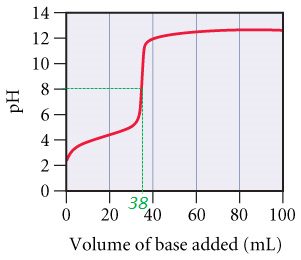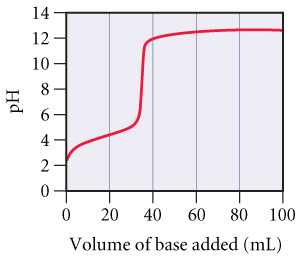# Problem: A 0.446-g sample of an unknown monoprotic acid was titrated with 0.105 M KOH and the resulting titration curve is shown in the figure.Determine the molar mass of the acid.

###### FREE Expert Solution

Recall that at the equivalence point of a titration:

Also, recall that moles = molarity × volume

$\overline{){{\mathbf{moles}}}_{{\mathbf{acid}}}{\mathbf{=}}{\left(\mathbf{MV}\right)}_{{\mathbf{base}}}}$

Volume at equivalence point = 38 mL KOH###### Problem Details

A 0.446-g sample of an unknown monoprotic acid was titrated with 0.105 M KOH and the resulting titration curve is shown in the figure.Determine the molar mass of the acid.